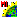# vsetflag.sml

###Download

#### Syntax Highlighing:

```comments, key words, predefined symbols, class members & methods, functions & classes
```
```# vsetflag.sml
clear();
vector V;
array numeric xPoints, yPoints;
numeric numPolys, numPoints;
GetOutputVector(V, "VectorToolkit");
# not going to use labels so tell it not to create qtree
VectorSetFlags(V, "NoLabelQTree");
# draw some boxes  - this will create polygons
# all closed lines are converted to polygons
xPoints = 0;
yPoints = 0;
xPoints = 100;
yPoints = 0;
xPoints = 100;
yPoints = 100;
xPoints = 0;
yPoints = 100;
xPoints = 0;
yPoints = 0;
VectorAddLine(V, 5, xPoints, yPoints);
# now add some points
numeric i;
for i = 1 to 4 {
VectorAddPoint(V, xPoints[i] + 150, yPoints[i] + 150);
}
numPolys = NumVectorPolys(V);
numPoints = NumVectorPoints(V);
print("number of polygons, points: ", numPolys, numPoints);
```